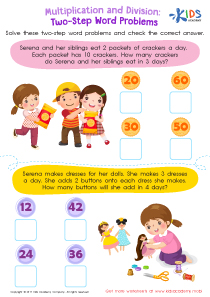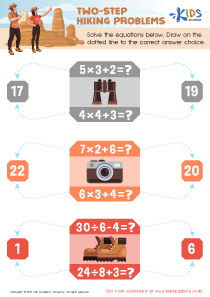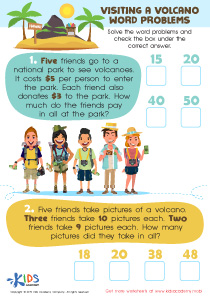# Math Lesson - 2 Step Word Problems - Drawing it Out, Grade 3

• ### Activity 1 / Two Step Word Problems WorksheetTake your child’s math skills up a notch by offering this fun and realistic two-step word problems worksheet for 3rd grade! By completing these word problems, kids learn to break down word problems into separate steps to solve tricky multiplication word problems on this free PDF worksheet!

• ### Activity 3 / Two-Step Hiking Problems WorksheetLearning math will be no smooth and easy task for your kids, as they will be introduced to new theories and problems every day. However, you can definitely do your best to make sure that their journey is easier for them by helping them with this worksheet. In the printout, there are three simple equations. Help your kids solve the equations, and then draw on the dotted line to the correct answer choice.

• ### Activity 4 / Visiting a Volcano Word Problems WorksheetWhen mathematical problems are presented in the form of sentences, these are called word problems. Word problems are meant to be solved just like regular number problems, but first, you must understand and interpret the sentence. In this worksheet, there are two short texts. Read the word problems to your kids and help them note down the numbers. Then, solve the word problems and check the box under the correct answer.

?